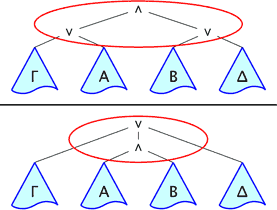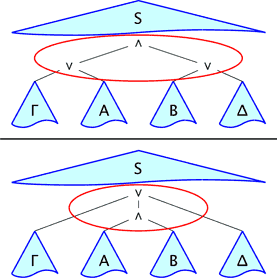Alessio Guglielmi's Research and Teaching / Deep Inference / Deep Inference in One Minute

## Deep Inference in One MinuteWe call 'shallow inference' the methodology behind the traditional formalisms of proof theory: Hilbert-Frege systems, natural deduction, sequent calculus, etc. In all these formalisms, inference rules can only be applied at the root of formulae (or sequents, or other structures). This rigidly constrains deduction, and a great deal of readily available information in formulae is not used.

The difference between Gentzen formalisms and deep inference ones is that in deep inference we compose proofs by the same connectives of formulae: if

 $$\Phi=\array{A\\ \Vert\\ B\\}$$ and $$\Psi=\array{C\\ \Vert\\ D\\}$$

are two proofs with, respectively, premisses $$A$$ and $$C$$, and conclusions $$B$$ and $$D$$, then

 $$\Phi\land\Psi=\array{A\land C\\ \Vert\\ B\land D\\}$$ and $$\Phi\lor\Psi=\array{A\lor C\\ \Vert\\ B\lor D\\}$$

are valid proofs with, respectively, premisses $$A\land C$$ and $$A\lor C$$, and conclusions $$B\land D$$ and $$B\lor D$$. Significantly, while $$\Phi\land\Psi$$ can be represented in Gentzen, $$\Phi\lor\Psi$$ cannot, or it only can with severe limitations.

As a consequence, in deep-inference formalisms, like the calculus of structures or open deduction, inference rules can be applied anywhere. This way, all the information is available to deduction.

 Shallow Inference Deep InferenceAbove there's the sequent-calculus inference $\frac{\Gamma,A\quad B,\Delta}{\Gamma,A\land B,\Delta} \quad.$ The part circled in red is the only one that can be inspected by the inference rule, and it can only occur at the root of the entire structure. Above there's the calculus-of-structures inference $\frac{S\{(\Gamma\lor A)\land(B\lor\Delta)\}} {S\{\Gamma\lor(A\land B)\lor\Delta\}} \quad.$ The inference rule can be applied anywhere, i.e., $$S$$ is an additional schematic variable.

Deep inference can be exploited in many ways, notably:

• to recover a De Morgan premiss-conclusion symmetry that is lost in Gentzen;
• to obtain new notions of normalisation in addition to cut elimination;
• to shorten analytic proofs by exponential factors compared to Gentzen;
• to obtain quasipolynomial-time normalisation for propositional logic;
• to express logics that cannot be expressed in Gentzen;
• to make the proof theory of a vast range of logics regular and modular;
• to get proof systems whose inference rules are local, which is usually impossible in Gentzen;
• to inspire a new generation of proof nets and semantics of proofs;
• to investigate the nature of cut elimination;
• to type optimised versions of the λ-calculus that are not typeable in Gentzen;
• to model process algebras;
• to model quantum causal evolution ...
• ... and much more.

If it's such a simple idea, why wasn't deep inference exploited before? Perhaps, the main reason is that deep inference breaks at their core the traditional normalisation techniques. For example, in a typical cut elimination step, one needs to deal with the following situation: $\frac{\dfrac{\strut\Gamma,A\quad\Gamma,B}{\strut\Gamma,A\land B} \quad \dfrac{\strut\bar A,\bar B,\Delta}{\strut\bar A\lor\bar B,\Delta}} {\strut\Gamma,\Delta} \quad.$ We know how to normalise this piece of proof because we know that the formulae $$A\land B$$ and $$\bar A\lor\bar B$$ are taken apart at their root by the shallow-inference rules. However, this is not true in the case of deep inference. This crucial piece of information is missing, so new techniques for normalisation need to be developed. This is far from trivial. For the calculus of structures and open deduction (which subsumes the calculus of structures), we developed fairly general, new techniques, which not only prove cut elimination but give us access to many other, very fine-grained normalisation notions, that are simply impossible in shallow inference.

2.5.2015 Alessio Guglielmiemail